# 自制操作系统09-不用main函数编程

1.环境

1.windows xp
2.trubo C 2.0

trubo c只需要几个文件，不需安装,如下图: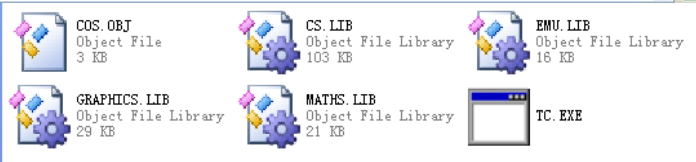```main(){
//printf("%x",main);
*(char far *)(0xb8000000+160*10+80)='a';
*(char far *)(0xb8000000+160*10+81)=2;
}```

2.破除main函数的魔咒

```f(){
*(char far *)(0xb8000000+160*10+80)='a';
*(char far *)(0xb8000000+160*10+81)=2;
}```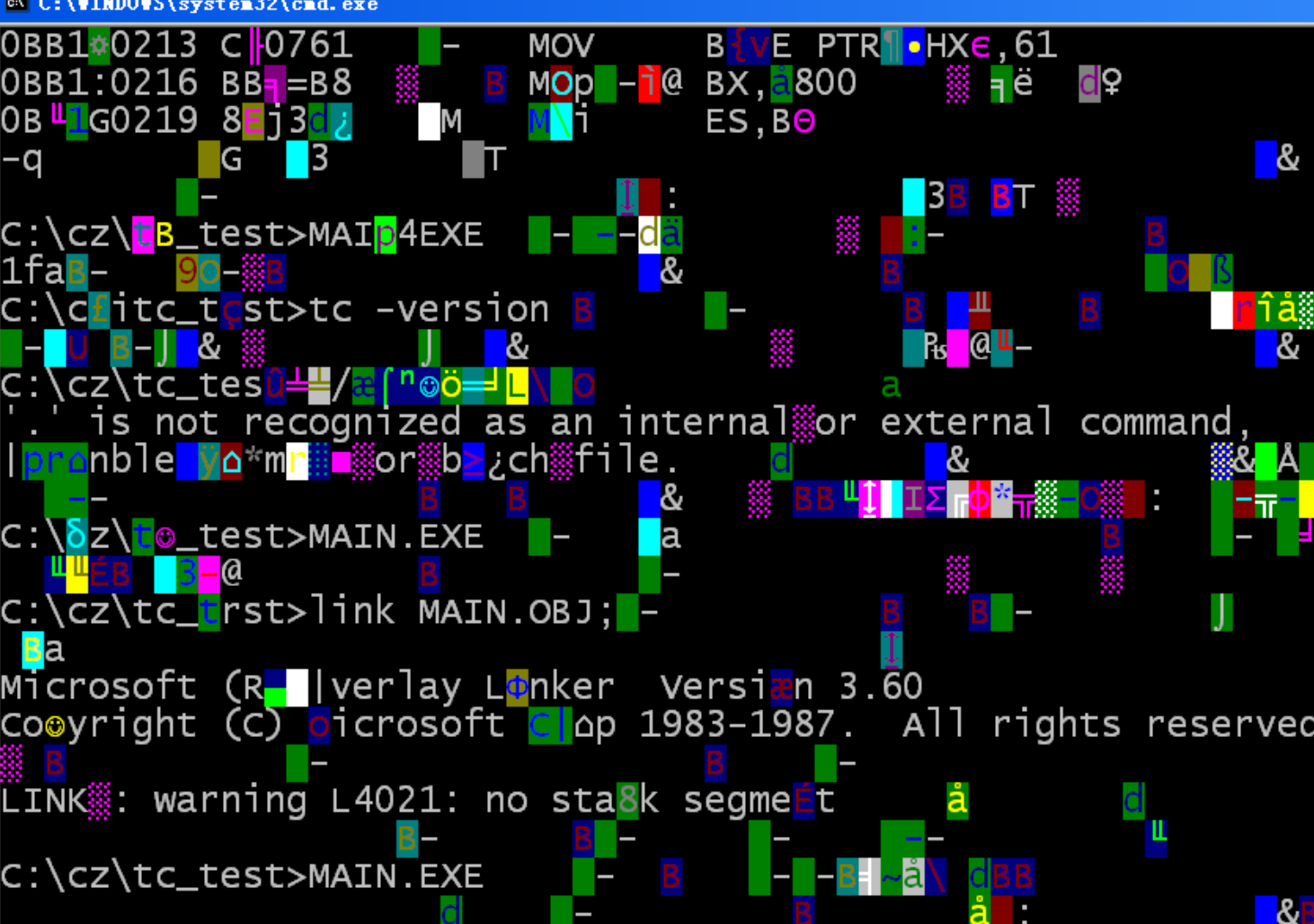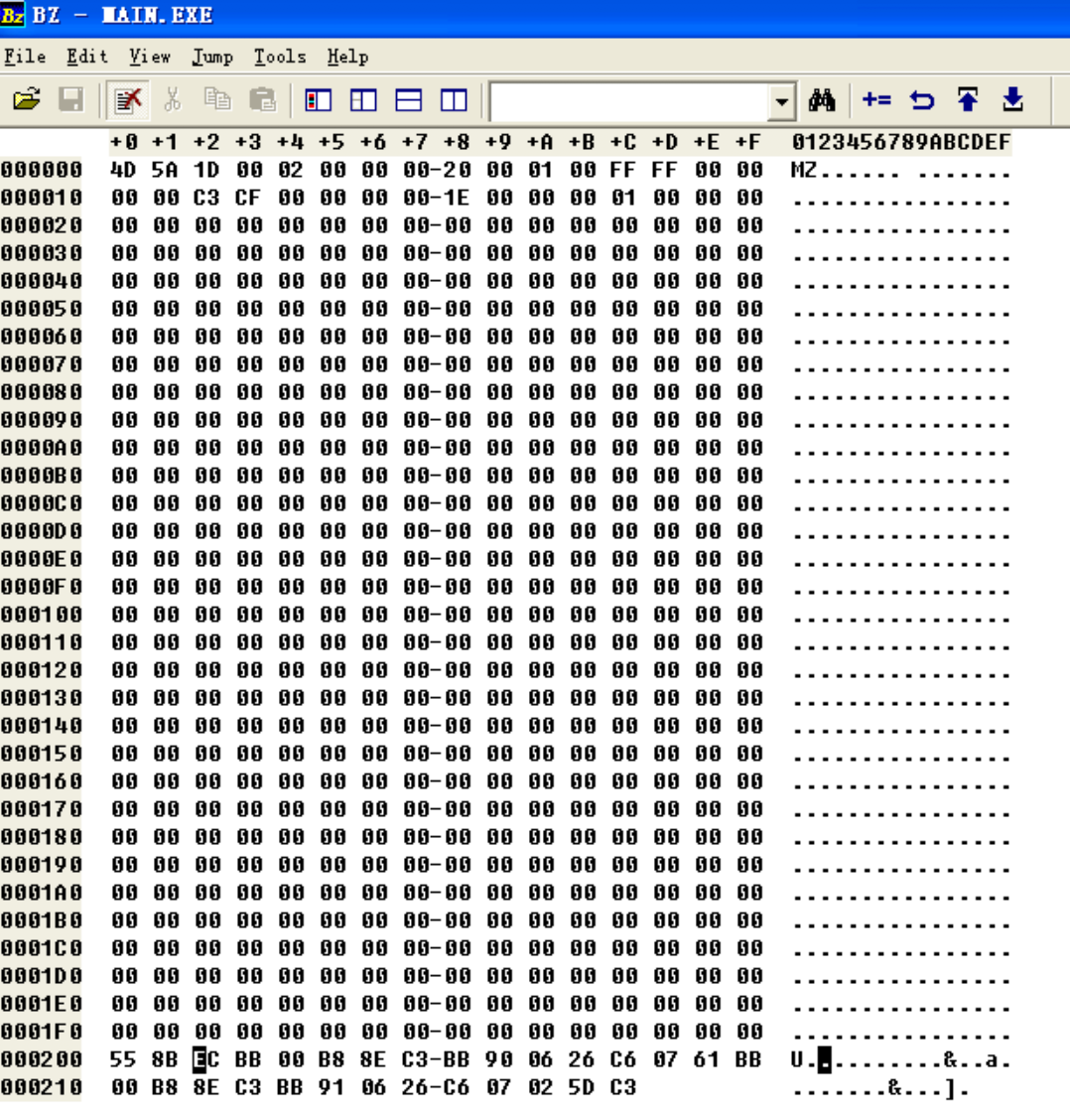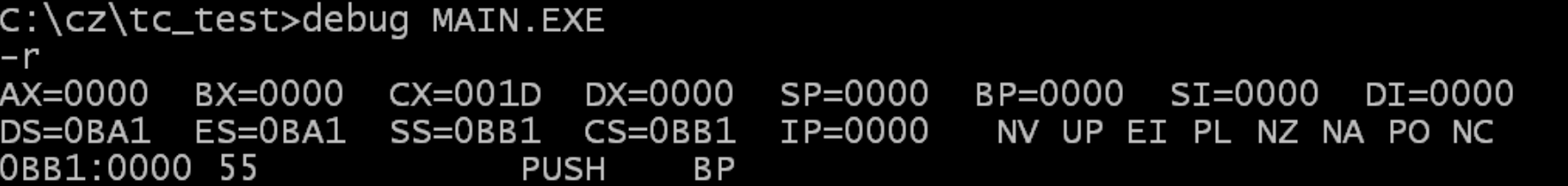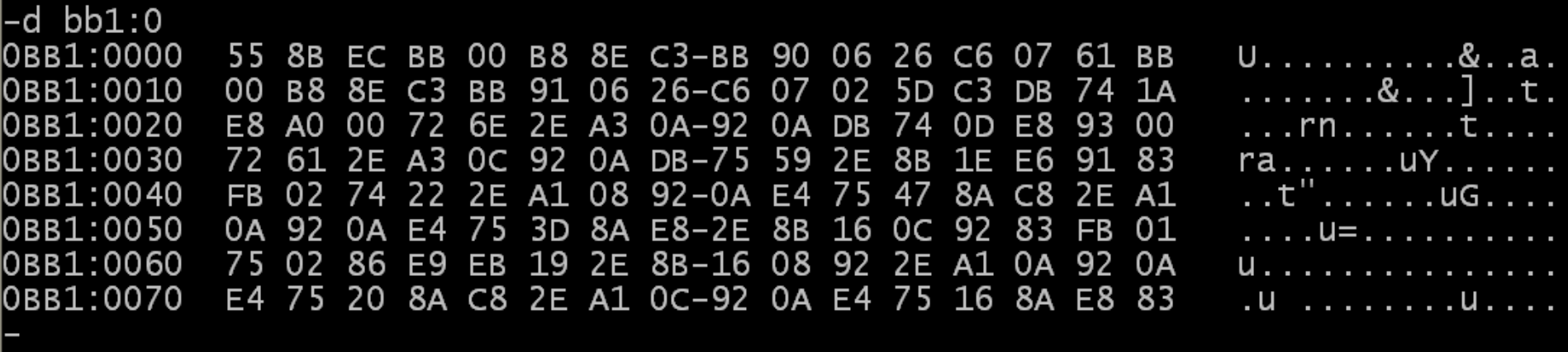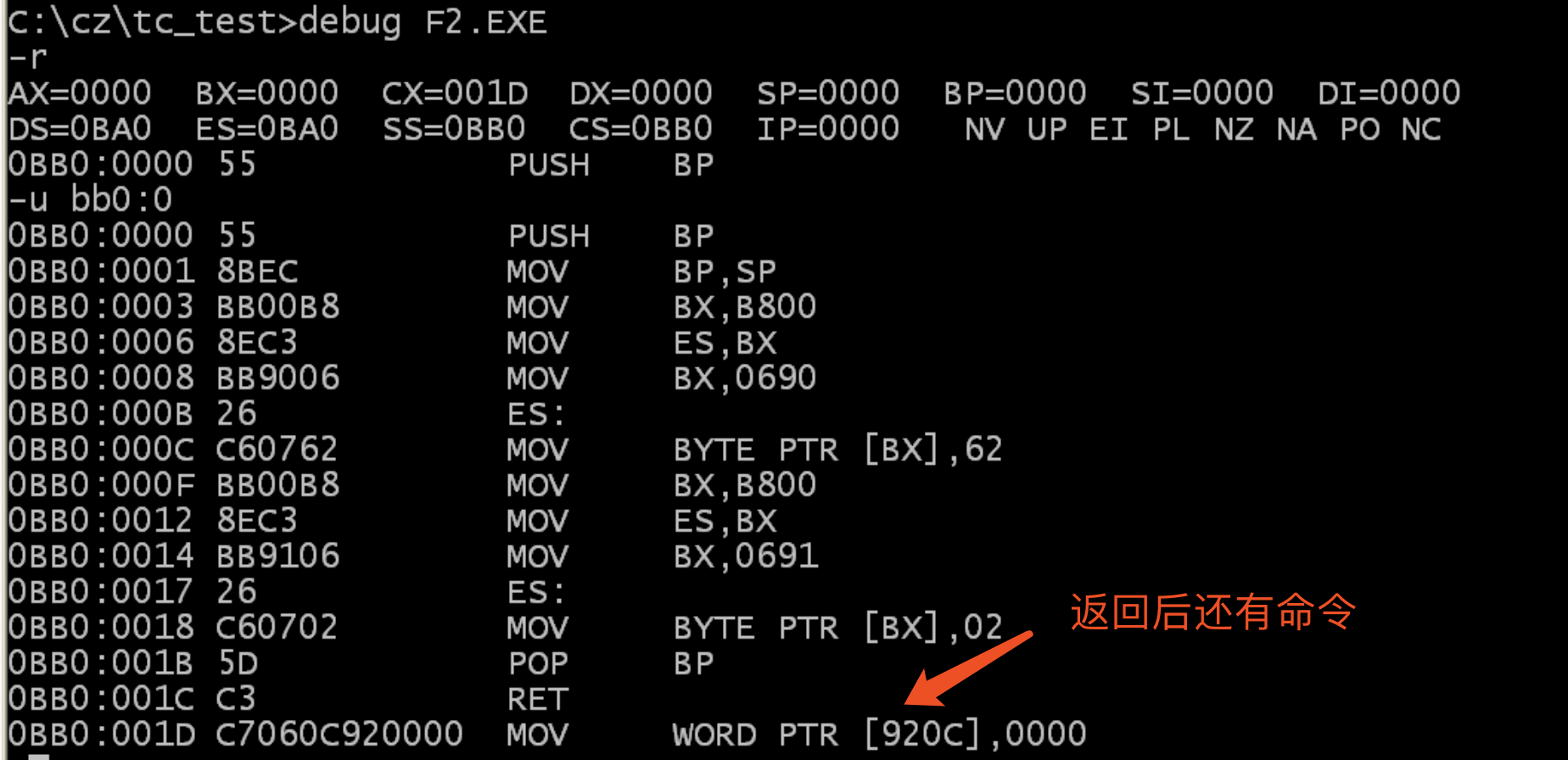mov ax,4c00h
int 21h

```assume cs:code
data segment
db 128 dup (0)
data ends

code segment
start:    mov ax,data
mov ds,ax
mov ss,ax
mov sp,128

call s

mov ax,4c00h
int 21h
s:
code ends

end start```

c0s.obj与f.obj的位置不能换，经实验生成的exe是严格按照这个顺序来生成可执行文件的，换了就无法正常运行。

3.讨论分析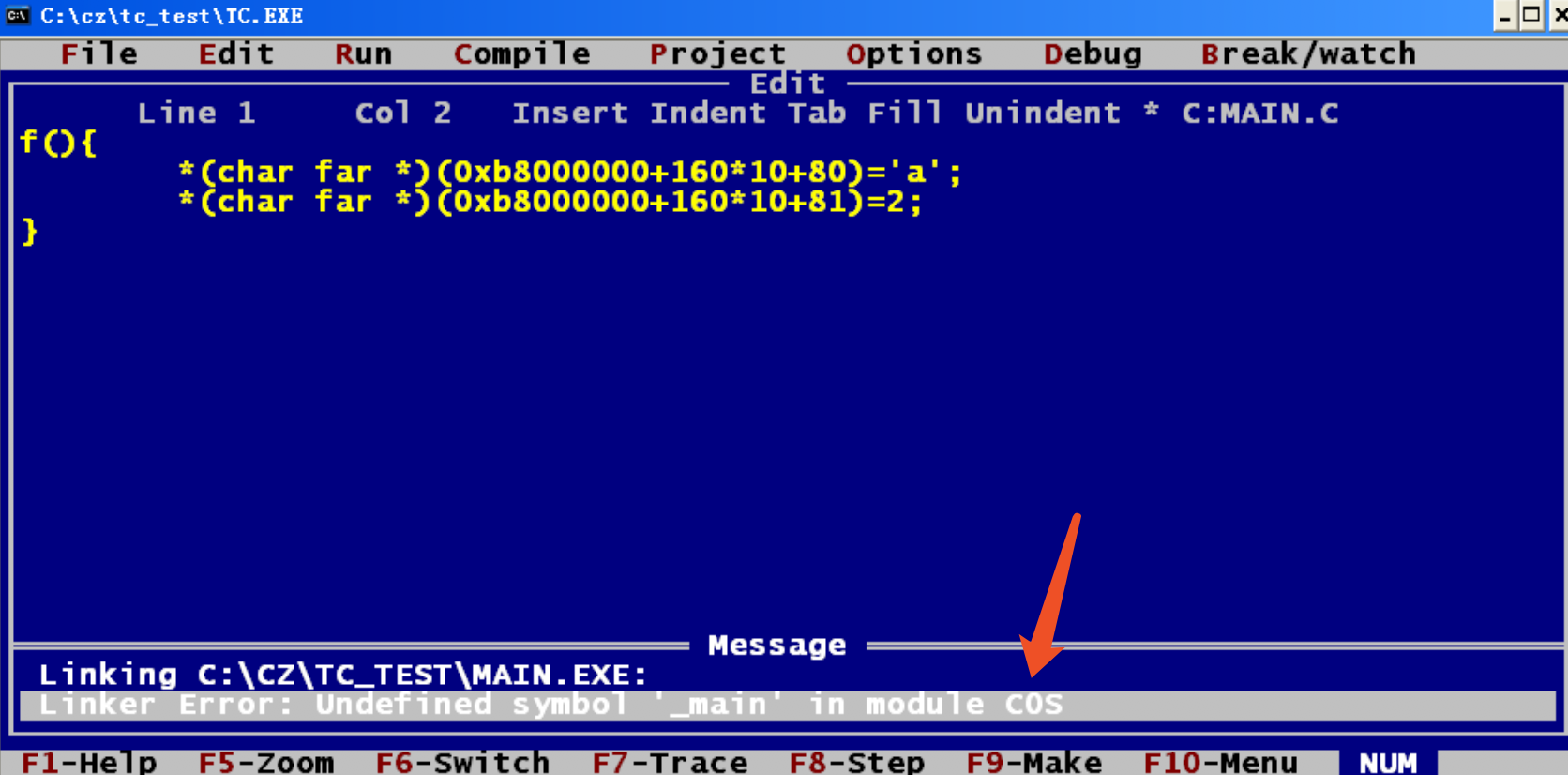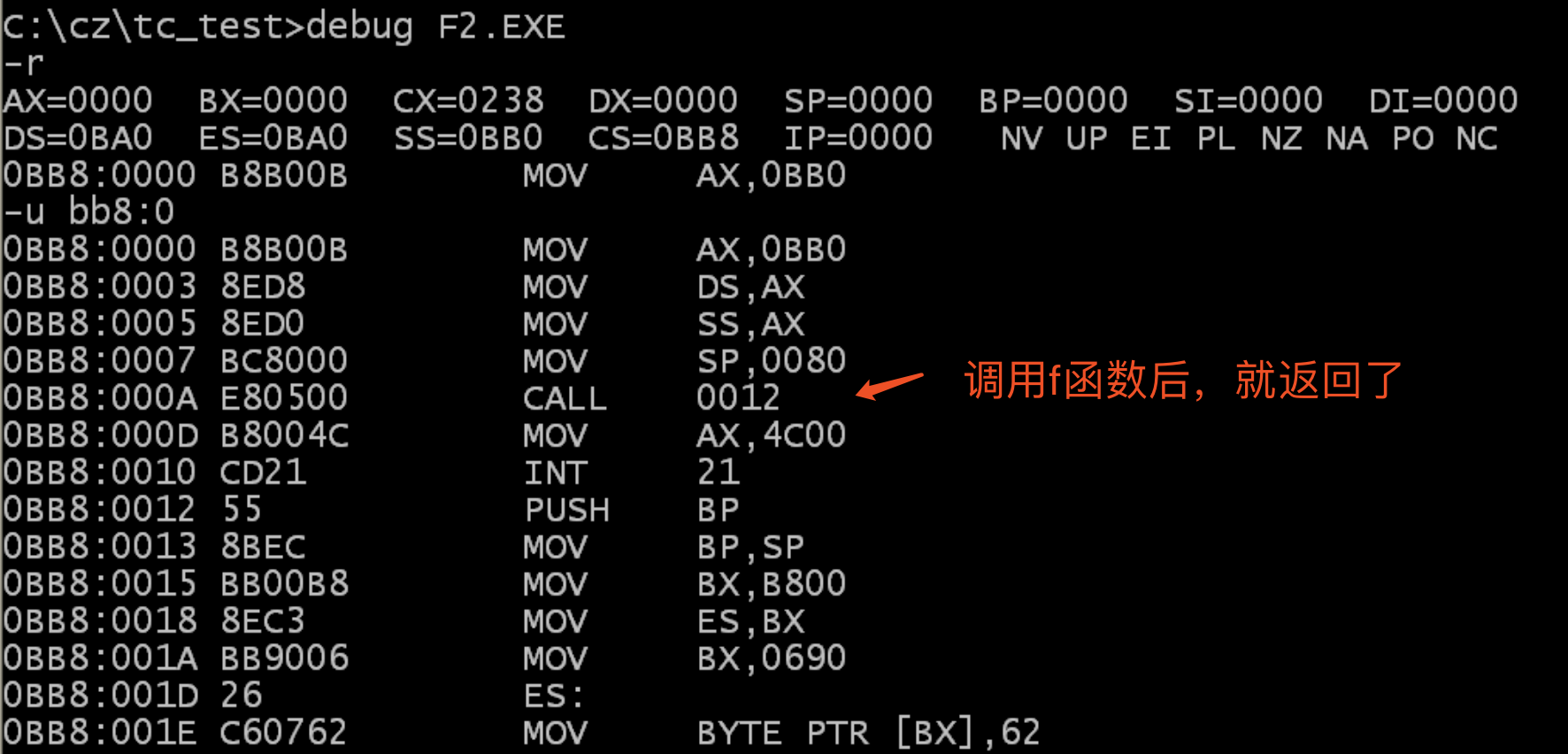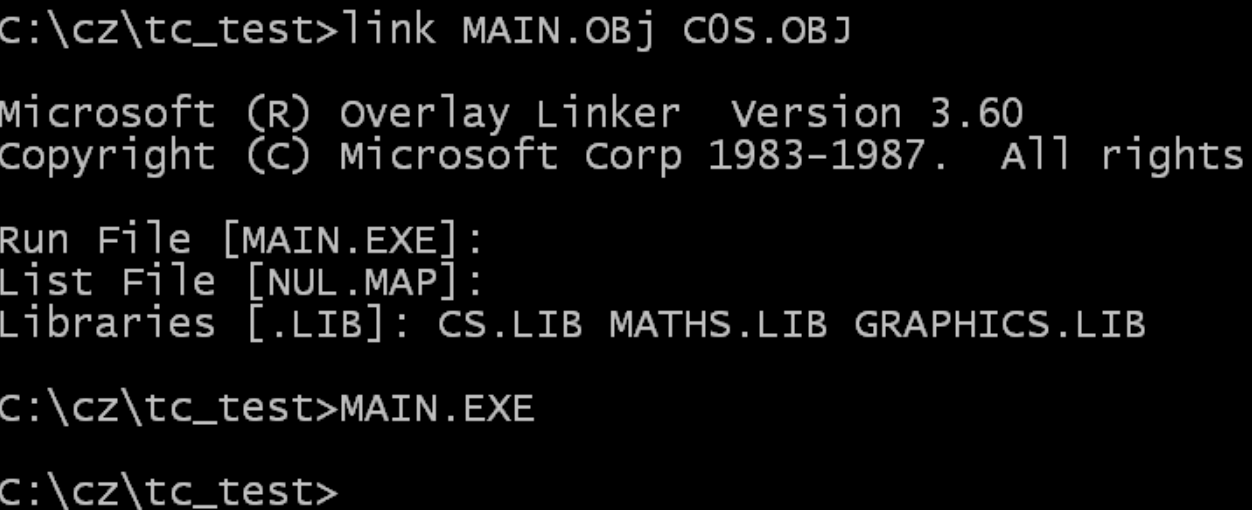### 相关阅读登录